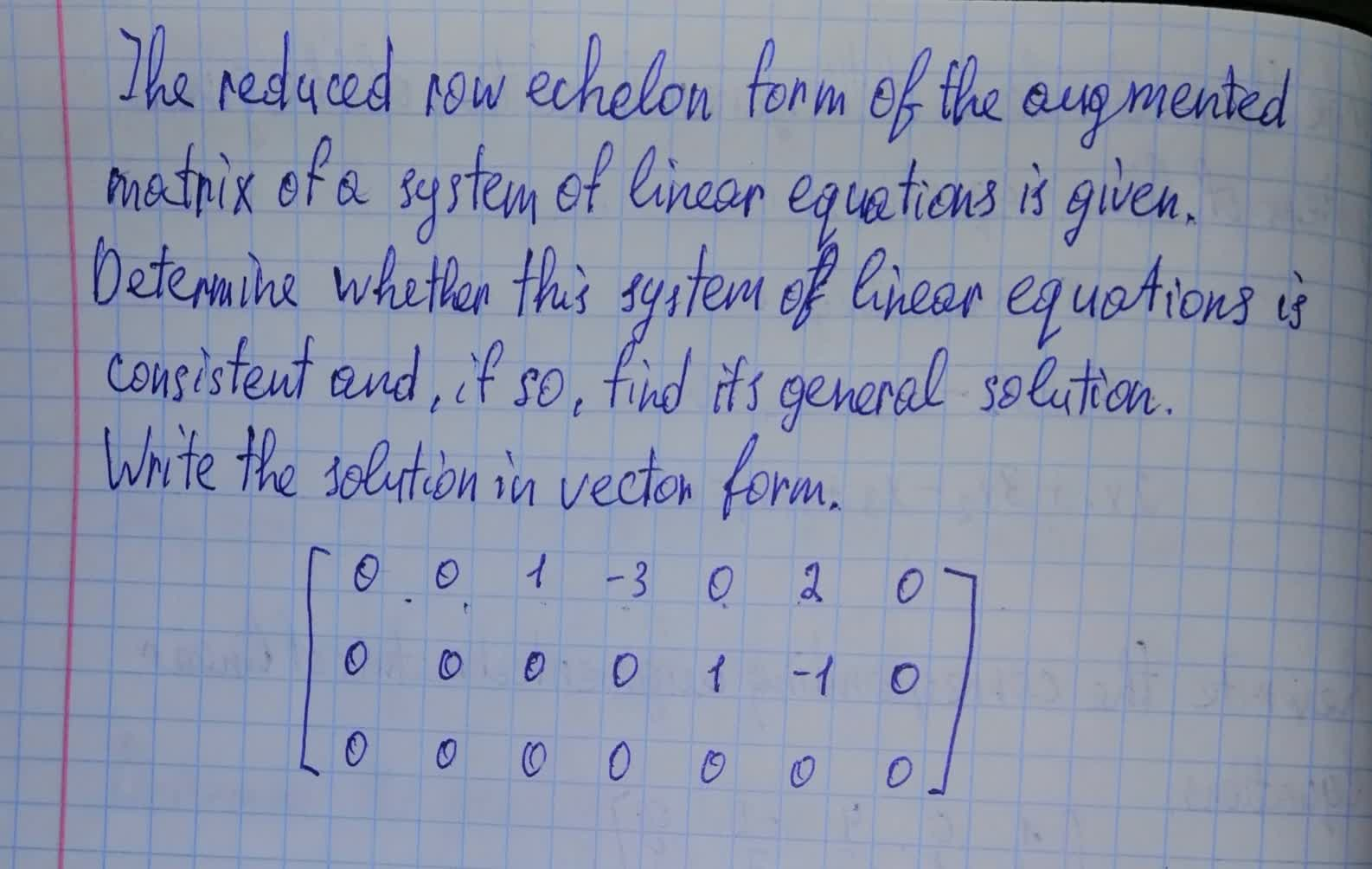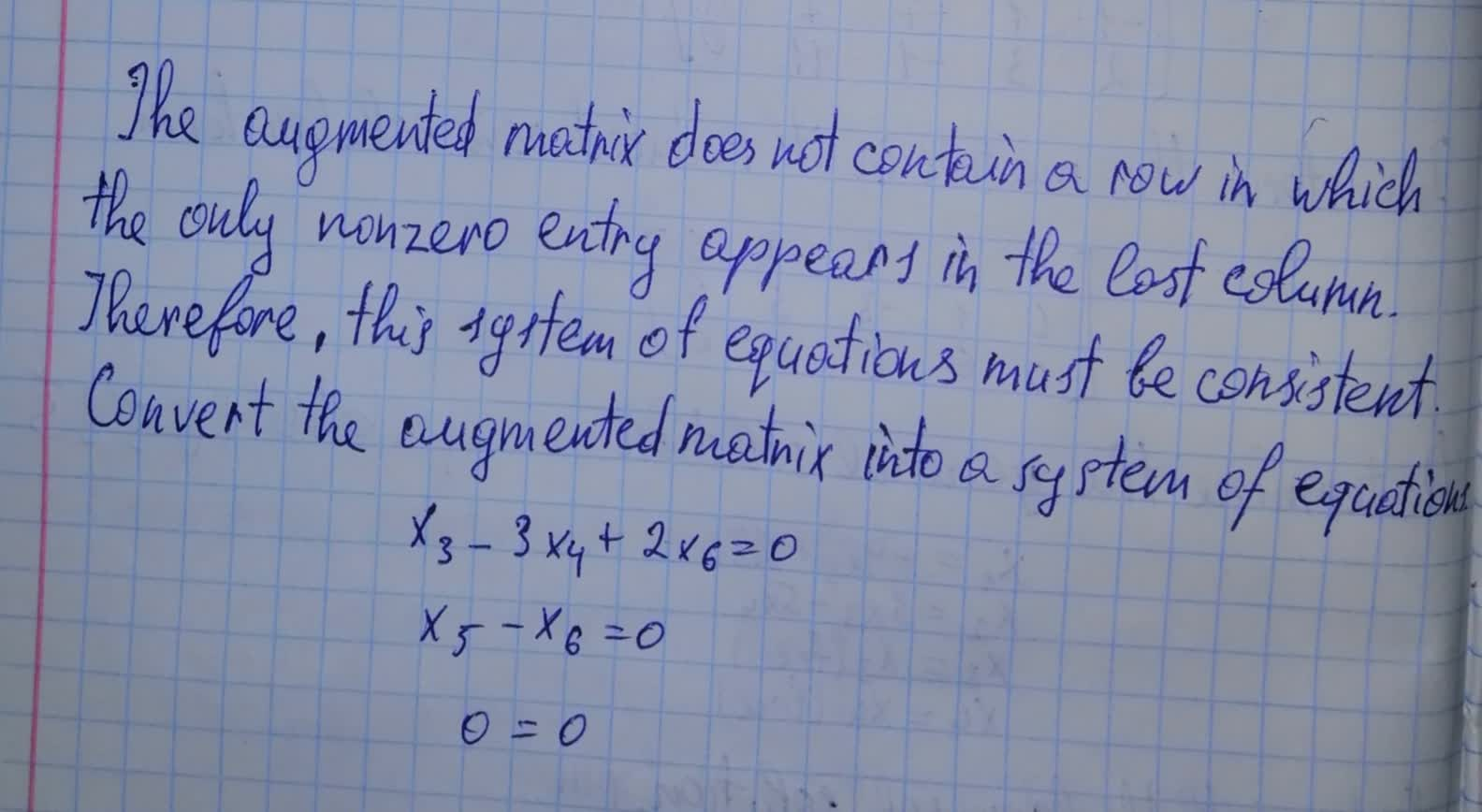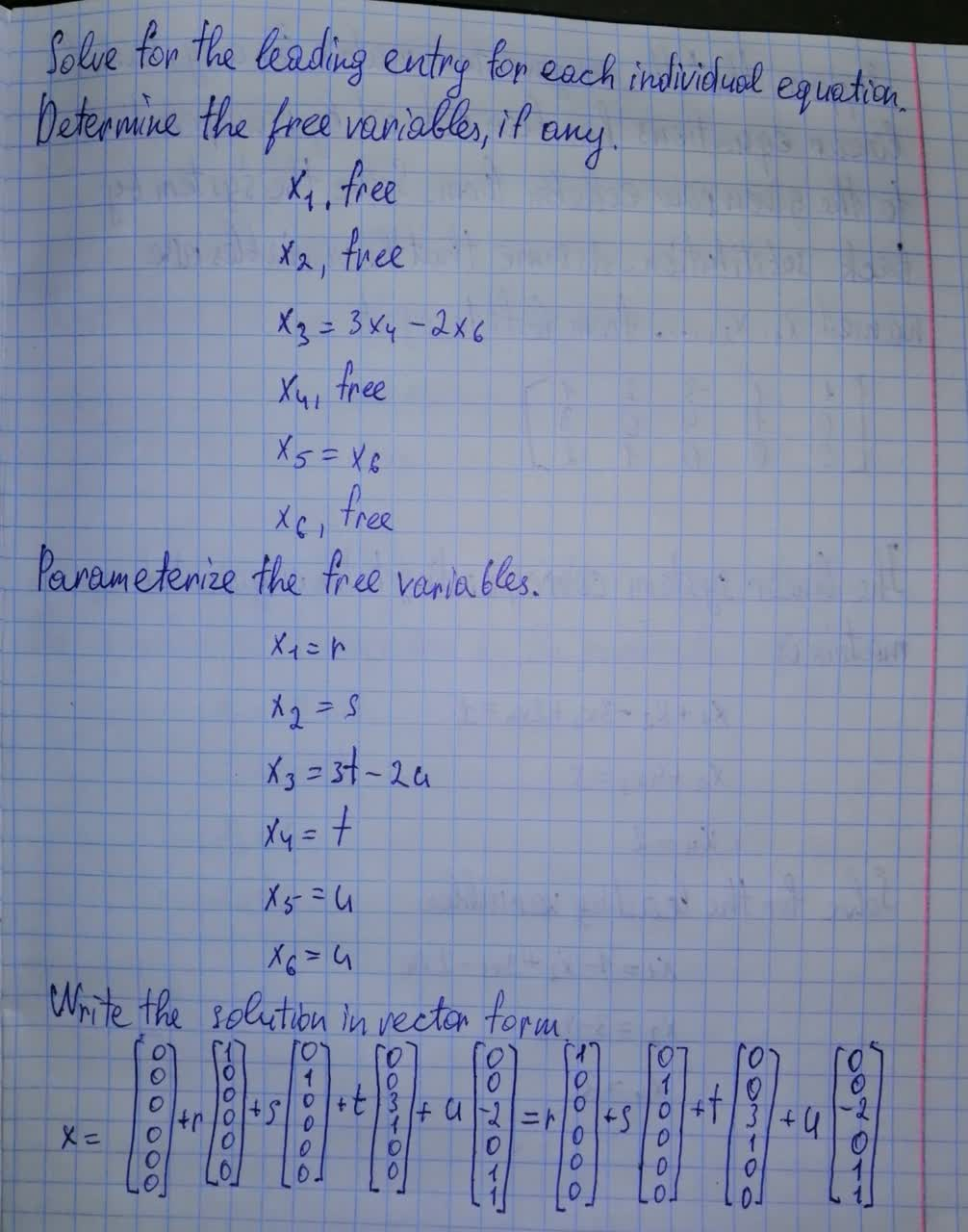Question# The reduced row form of the matrix of a system of linear equations is given.\begin{bmatrix}0&0&1&-3&0&2&0 \\0&0&0&0&1&-1&0\\0&0&0&0&0&0&0\end{bmatrix}

Forms of linear equations
ANSWEREDThe reduced row echelon form of the augmented matrix of a system of linear equations is given. Determine whether this system of linear equations is consistent and, if so, find its general solution. $$\begin{bmatrix} {0}&{0}&{1}&-{3}&{0}&{2}&{0}\\ {0}&{0}&{0}&{0}&{1}&-{1}&{0}\\ {0}&{0}&{0}&{0}&{0}&{0}&{0}\\ \end{bmatrix}$$